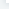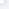# 用R语言绘制直方图――hist函数

：2018-07-08 10:58:34

hist(x, breaks = "Sturges",
freq = NULL, probability = !freq,
include.lowest = TRUE, right = TRUE,
density = NULL, angle = 45, col = NULL, border = NULL,
main = paste("Histogram of" , xname),
xlim = range(breaks), ylim = NULL,
xlab = xname, ylab,
axes = TRUE, plot = TRUE, labels = FALSE,
nclass = NULL, warn.unused = TRUE, …)

（1）x

（2）breaks

①可以是一个向量给出直方图中每个区间的断点；

②可以是一个函数用于计算每个断点的向量；

③用于表示区间数的一个数字；

④一个字符串用于给出计算区间数所使用的算法；

⑤一个用于计算区间数的函数。

（3）freq

（4）probability

（5）include.lowest

（6） right

（7）density

（8）angle

（9）col

（10）border

（11）main, xlim, ylim, xlab, ylab

（12）axes

（13）labels

（1）绘制19名学生的身高频率直方图。

`Height<-c(144,166,163,143,152,169,130,159,160,175,161,170,146,159,150,183,165,146,169)hist(Height,col="lightblue",border="red",labels=TRUE,ylim=c(0,7.2)) `

（2）hist(Height,breaks=12,freq=FALSE,density=10,angle=60)

（3）

data <- c(rep(1, 10), rep(2, 5), rep(3, 6))
hist(data, breaks = c(0.5, 1.5, 2.5, 3.5))

## 相关文章